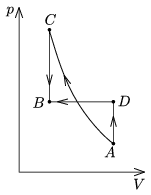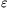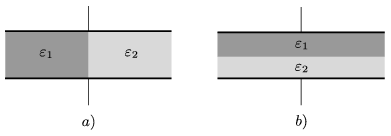Mathematical and Physical Journal
for High Schools
Issued by the MATFUND Foundation
 Already signed up? New to KöMaL?

# KöMaL Problems in Physics, March 2009

Show/hide problems of signs:## Problems with sign 'M'

Deadline expired on April 14, 2009.

M. 295. Drop a pebble into a container filled with water. Measure the greatest horizontal distance to which the water splashes as a function of the speed at which the pebble hits the water. Carry out the experiment with at least two pebbles which have different size.

(6 pont)

statistics## Problems with sign 'P'

Deadline expired on April 14, 2009.

P. 4144. Using simple equipment, how can you measure the mass of an object which is greater than 120 kg, with a scale which can measure maximum 120 kg?

(3 pont)

statistics

P. 4145. A passenger train passes a railroad-crossing at a speed of 72 km/h, and it blows a whistle at a frequency of 170 Hz. What is the pitch interval which is sensed by an observer standing next to the railroad-crossing?

(4 pont)

statistics

P. 4146. One end of a compressed spring is attached to a wall, and the other is attached to an object which can slide along a rough horizontal surface. The initial compression of the spring is 24 cm, and the system is left alone in this position. When the spring is in the unstretched position the mechanical energy of the system is half of the original energy. How far can the object move from the initial position?

(4 pont)

statistics

P. 4147. Will the energy of air in a cold room change when a stove in the room is heated?

(3 pont)

statistics

P. 4148. A sample of ideal gas is taken from state A to state B in two different ways. The two states has the same temperature.a) First it is compressed adiabatically (AC), and then it is cooled down such that its volume remains constant (CB);

b) First it is heated at constant volume (AD), then it is compressed isobarically (DB).

In which case do we perform less work? At state C or at state D will the temperature of the gas be greater?

(5 pont)

statistics

P. 4149. A 25 cm-long simple pendulum is hanging vertically inside a small car which is at rest. The mass of the bob is 2 grams, its charge is 5.10-8 coulomb. The vehicle is in uniform electric field, and then it will travel in this field. The electric field points vertically upward and its magnitude is 400 kN/C. The vehicle is started at an acceleration of 4.5 m/s2. Air resistance is negligible.

a) Find the maximum speed of the bob with respect to the vehicle.

b) What must the strength of the cord be in order not to be broken during the motion.

(5 pont)

statistics

P. 4150. One half of the space between the two plates of a parallel plate capacitor is filled with a dielectric of permittivity1 and the other half is filled with another dielectric of permittivity2, first as shown in figure a) and second as shown in figure b). In which case will the capacitance of the capacitor be greater?(4 pont)

statistics

P. 4151. Can an electric field be created such that the electric field lines are parallel but their density is not constant

a) in vacuum,

b) in a dielectric?

(5 pont)

statistics

P. 4152. The filament of an incandescent lamp of power 100 W is made of Tungsten. The operation temperature of the lamp is 2400 K. Consider the filament a black body and give an estimation for the surface area, the cross section, and the length of the filament.

(5 pont)

statistics

P. 4153. When the nucleus of a Beryllium atom is hit by a proton, the nucleus of a Lithium atom and anparticle were initiated. Determine the energy liberated in the reaction.

(4 pont)

statistics